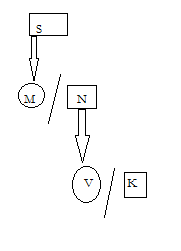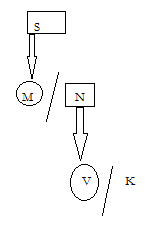# SSC CGL 2018 Practice Test Papers | Reasoning Ability (Day-4)

Dear Aspirants, Here we have given the Important SSC CGL Exam 2018 Practice Test Papers. Candidates those who are preparing for SSC CGL 2018 can practice these questions to get more confidence to Crack SSC CGL 2018 Examination.

[WpProQuiz 2664]

Click “Start Quiz” to attend these Questions and view Explanation

1. A person starts from ground and travels 4 km towards west and takes left turn and travel 5 km and takes left travel 2 km and takes left travel 8 km to reach office. In which direction now he was going?

A) South

B) North

C) East

D) West

1. Find the number in the missing number series.

2   ?   -2   7   -20

A) 4

B) 6

C) 3

D) 1

1. There are twenty students in the class. Raj sits 10th from the left and Ramu sits 5th from the right. How many persons sit between Raj and Ramu?

A) 5

B) 6

C) 7

D) 8

1. Statement :

All silver are tree

Some hotel are hindi

No tree is hindi

Conclusion :

1. All hotel are hindi
2. Some trees are silver
3. All hotel are hindi is a possibility

A) only one follows

B) only 2 and 3 follows

C) only 3 follows

D) none follows

5.INDIA is coded as FNISN, JAPAN is coded as SFUFO, then how CRACK is coded as

A) PHEWH

B) PHFWH

C) PHBWH

D) PHDWH

1. If H 17 S 6 J 15 A 24.Then

M ? N 11 P 9 R 7

A) 12

B) 14

C) 16

D) 18

1. If + means ÷, – means × , ÷ means – , × means + then

(25 × 50) + 5 x 30 ÷ 10?

A) 50

B) 35

C) 53

D) 44

1. Directions: A+O means A is the mother of O

A-B means A is the father of B

A × D means A is the wife of D

A ÷ C means A is the sister of C

If S- M × N-V ÷ K then which statement is true

A) S is the mother of M

B) S is the mother of K

C) M is the mother of V

D) K is the son of M

9.How K is related to M

If S-M ×N- V ÷K is true

A) K is the Son of M

B) Can’t be determined

C) K is the daughter of M

D) K is the Nephew of M

10.Piyush Goyal : Railway : : ? : Food Processing

A ) Uma Bharathi

C) Suresh Prabhu

Explanation: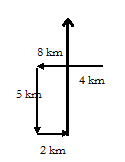Explanation: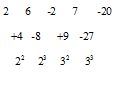Explanation: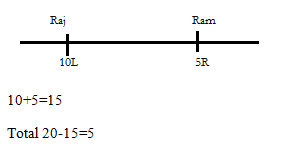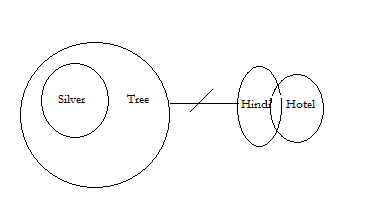Explanation :

CRACK

K+5 =P

C+5 = H and so on

Explanation :

From reverse (z=1, y=2….)

H =19 -2 = 17

M=14-2=12

(25+50)/5+30-10=35